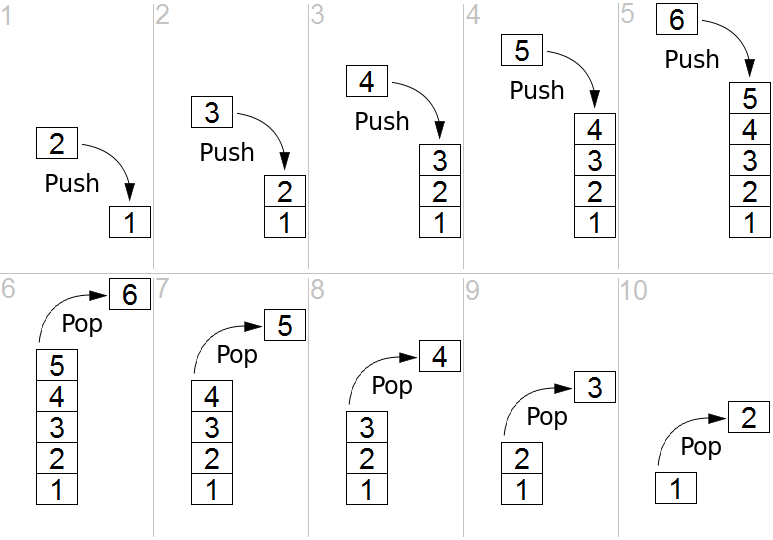# Check whether the given parenthesis filled string is balanced? (Java)

In this Java article, you will learn how to check if the parenthesis filled string is balanced or not in JAVA. Here we have provided a Java program on check parenthesis filled string is balanced or not in JAVA

## Check if the given parenthesis containing string is balanced or not

#### STACK using JAVA

STACK or LIFO (last in, first out), according to Wikipedia, is an abstract data type that serves as a collection of elements in computer science, with two principal operations:

• push: adds an element to the collection
• pop: removes the last element that was addedWhen the following conditions are true, then we might call the given parenthesis containing string as balanced.

• If it’s an empty string
• If B and A are correct, BA is correct,
• If B is correct, (B) and {B} and [B] are also correct.

Examples are:

1. Balanced strings : “(){}”, “[({})]”, “({[])})”,{()}
2. Unbalanced strings : “{}([“, “([)}”, “[[{“, “}({” etc.

Now, we will see how to determine if it is balanced or not using Java.

Format of Input

The input file must have multiple lines, each having a single non-empty string. You should read input till end-of-file.

Format of Output

For the string in each input line, print corresponding output as ‘true’ if the string is balanced, else print ‘false’.

### Program: Check parenthesis filled string is balanced or not in JAVA

```//JAVA8

import java.io.InputStream;
import java.util.Vector;
import java.io.OutputStream;
import java.util.Stack;
import java.io.PrintWriter;
import java.io.IOException;
import java.util.StringTokenizer;
public class Solution {
public static void main(String[] args) {
InputStream inputStream = System.in;
OutputStream outputStream = System.out;
PrintWriter out = new PrintWriter(outputStream);
solver.solution(1, in, out);
out.close();
}
}
public void solution(int testNumber, InputReader in, PrintWriter out) {
String str;
try {
Stack<Character> s = new Stack<>();
boolean ans = true;
for (int i = 0; i < str.length(); i++) {
Character my_char = str.charAt(i);
if (my_char == '(' || my_char == '{' || my_char == '[') {
s.push(my_char );
} else if (my_char == ')') {
if (!s.isEmpty() && s.peek() == '(') {
s.pop();
} else {
ans = false;
}
} else if (my_char == '}') {
if (!s.isEmpty() && s.peek() == '{') {
s.pop();
} else {
ans = false;
}
} else if (my_char == ']') {
if (!s.isEmpty() && s.peek() == '[') {
s.pop();
} else {
ans = false;
}
}
}
out.println(ans && s.isEmpty());
}
} catch (IOException e) {
}
}
}
public StringTokenizer tokenizer;

tokenizer = null;
}
}```

Input

```{}()
({()})
{}(
[]```

Output

```true
true
false
true```

Hope this tutorial helped you to clear your doubts and solve your problem.

### 2 responses to “Check whether the given parenthesis filled string is balanced? (Java)”

1.Lutus says:

public class CountParens
{
public static void main(String args[])
{
for(String arg : args) {
int lp = arg.replaceAll(“[^(]”, “”).length();
int rp = arg.replaceAll(“[^)]”, “”).length();
String result = String.format(
“String \”%s\” : lp = %d rp = %d rp-lp = %d”
, arg, lp, rp, rp-lp);
System.out.println(result);
}
}
}

Example:

\$ java CountParens “(((( try this out ))))” “(())())”
String “(((( try this out ))))” : lp = 4 rp = 4 rp-lp = 0
String “(())())” : lp = 3 rp = 4 rp-lp = 1

I think this would be much better. Using stack will just make it more complicated

2.aj says:

String “)(“: lp = 1 rp = 1 rp-lp = 0